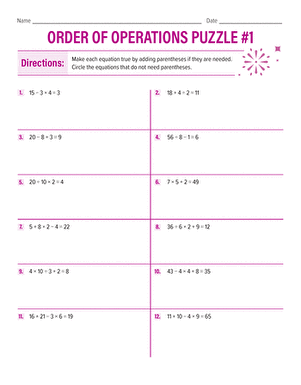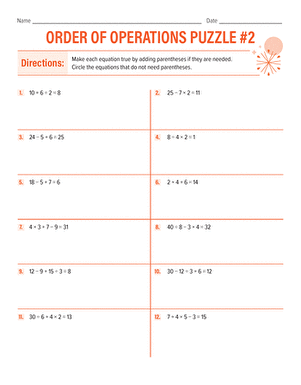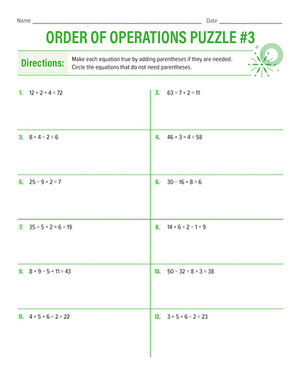Learning Library

# Order of Operations Puzzles

In these cool order of operations puzzles, 4th graders put their logic to the test and learn to think algebraically by figuring out how to use parentheses to make each equation a true statement.### Order of Operations Puzzle

Use the rules for the order of operations to write in the missing parenthesis that make each equation true.### Order of Operations Puzzle 2

Practise beginning algebra with an order of operations puzzle! Put in the missing parentheses to make each equation true.### Order of Operations Puzzle 3

Practise the rules of the order of operations with a logic puzzle! Write in the missing parentheses to make each equation true.

Create new collection

0

### New Collection>

0Items

What could we do to improve Education.com?